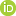This is a Preprint and has not been peer reviewed.

##### Authors

Robert Myhill, James Connolly

##### Abstract

A large class of solid solution models are built on the premise that exchange of chemical species takes place on a finite number of unique sites, and that the thermodynamic properties of the solution are a function of the proportions of species occupying each of the sites. The site-occupancy spaces spanned by such models are geometrically equivalent to convex polytopes, n-dimensional generalisations of polygons and polyhedra. The endmembers of these solid solutions correspond to the vertices of the polytopes.We present a set of mathematical tools based on this geometrical equivalence which aid the creation and manipulation of solution models. Vertex enumeration methods can be used to compute the total set of endmembers in
a solution from the valences of the species occupying each site and the total charge of the species not involved in site exchange. The number of independent endmembers constructed in this way is equal to n site-species − n sites + c, where n site-species is the total number of potential site-species occupancies in the solution and n sites is the number of distinct sites. If charge-balance constraints are active, c = 0, otherwise c = 1.
We also present the linear algebra required to transform solution model parameterisations between different independent endmember bases. The same algorithms can also be used to derive macroscopic endmember interactions from microscopic site interactions. This algebra is useful both in the initial design of solution models, and when making thermodynamic calculations in restricted chemical subsystems.

##### DOI

https://doi.org/10.31223/osf.io/fhwjy

##### Subjects

Chemistry, Earth Sciences, Materials Chemistry, Mineral Physics, Physical Sciences and Mathematics

##### Dates

Published: 2019-12-04 16:44